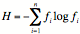Basic Descriptive Statistics

Types of Biological Data:

Any observation or experiment in biology involves the collection of information, and this may be of several general types:

Data on a Ratio Scale:  Consider measuring heights of plants. Then the difference in height between a 20 cm tall plant and a 24 cm tall plant is the same as that between a 26 cm tall plant and a 30 cm tall plant. These data have a "constant interval size". They also have a true zero point on the measurement scale, so that ratios of measurements make sense (for example, it makes sense to state that one plant is 3 times as tall as another). A measurement scale that has constant interval size and a true zero point are called "ratio scales". Ex: weights (mg, kg), length (cm, m), volumes (cc, cu m), lengths of time (s, min).

Data on an Interval Scale:  Measurements with an interval scale but having no true zero point are of this type. Examples are temperature measured in Celcius or Farenheit - it makes no sense to say that 40 degrees is twice as hot as 20 degrees. Absolute temperatures are measured on a ratio scale though.

Data on an Ordinal Scale:  Data consisting of a ranking or ordering of measurements are on an ordinal scale. Examples would be rankings based on size of objects, how fast an individual is, how deep of an orange color a shirt is, etc. In some cases (e.g. size) there may be an underlying ratio scale, but if all that is provided is a ranking of individuals (e.g. you are only told that tomato genotype A is larger than tomato genotype B, not how much larger), there is a loss of information in just being given the ranking on an ordinal scale. Quantitative comparisons are not possible on ordinal scale (how can one say that one shirt is half as orange as another?).

Data on Nominal Scale:  When a measurement is classified by an attribute that it has, rather than by a quantitative, numerical measurement, then it is on a nominal scale (male or female; genotype AA, Aa or aa; in the taxa Pinus or in the taxa Abies; etc.). Often, these are called categorical data because you categorize the data elements according to what category it is in.

Continuous vs. Discrete Data:  When a measurement can take on any conceivable value along a continuum, it is called continuous. Weight and height are continuous variables. When a measurement can only take on one of a discrete list of values, it is discrete. Number of arms on a starfish, number of leaves on a plant, number of eggs in a nest are all discrete measurements.

Frequency Distributions:

When making repeated measurements from some experiment (e.g. measuring how many white blood cells are in a 1 ml sample of whole blood), it is typical to summarize the data pictorially by making a bar chart to display how frequently each count arises. Such graphs can be made for nominal data (the percentages of males versus females feeding female pups in meerkats -  see Fig 1 E in paper by Clutton-Brock et al.), ordinal data (the percentages of Juveniles, Subadults, yearlings and adults

contributing to babysitting in meerkats - see Fig 1 A in paper by Clutton-Brock et al.), discrete data (e.g. the frequency of meerkat litters of sizes 1, 2, 3, etc. pups), grouped discrete data (e.g. the numbers of white blood cells in a 1 ml whole blood sample, grouped by 0-100 cells, 101-200 cells, 201-300 cells, etc.), or continuous data, in which case we call the bar graph a histogram (weights of individuals in the room, grouped by 5 kg increments for example).

In histograms, one either shows the range of the continuous values associated with each bar, or else if giving a single number it is the midpoint of the interval covered by that bar. Generally, equal size intervals are used in histograms.

Summary Descriptive Statistics of Datasets:

Just as there is a loss of information in going from a list of observations to a histogram, any time a data set is summarized by statistical information about it, there is a loss of information. That is, given the summary statistics, there is no way to recover the original data. Basic summary statistics may be grouped as:

(i) measures of central tendency (giving in some sense the central value of a data set); and

(ii) measures of dispersion (giving a measure of how spread out that data set is).

Measures of central tendency:

Arithmetic mean (the average)

If the data collected as a sample from some set of observations have values

x1, x2 , ... , xn  then the mean of this sample is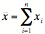The median is the middle value - half the data fall above this and half below. In some sense this supplies less information than the mean since it considers only the ranking of the data, not how much larger or smaller the data vales are. But the median is less affected than the mean by "outlier" points (e.g. a really large measurement or data value that skews the sample). The LD 50 is an example of a median - the median lethal dose of a substance (half the individuals die after being given this dose, and half survive). For a list of data x1, x2 , ... , xn  to find the median, list these in order from smallest to largest. If n is odd, the median is the number in the 1+ (n-1)/2 place on this list. If n is even, the median is the average of the numbers in the n/2 and 1+(n/2) positions on this list.

Quartiles arise when the sample is broken into 4 equal parts (the right end point of the 2nd quartile is the median), quintiles when 5 equal parts are used, etc.

The Mode is the most frequently occurring value (or values - there may be more than one) in a data set. A typical description of a biological data set is that it is unimodal (meaning that it has a single “peak” value), bimodel (it has two peaks), etc. Here

The Midrange is the value half-way between the largest and smallest values in the data set. So if xmin and xmax are the smallest and largest values in the data set then the Midrange is xmin + (xmax-xmin)/2

The geometric mean of a set of n data is the nth root of the product of the n data values.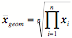The geometric mean arises as an appropriate estimate of growth rates of a population when the growth rates vary through time or space. It is always less than the arithmetic mean (it is equal to it if the data all have the same value).

The harmonic mean is the reciprocal of the arithmetic mean of the reciprocals of the data.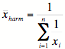It also arises in some circumstances as the appropriate overall growth rate when rates vary.

Measures of dispersion:

Range - this is the largest minus the smallest value in the data set: xmax-xmin . This doesn't account in any way for the manner in which data are distributed across the range.

The variance is the mean sum of the squares of the deviations of the data from the arithmetic mean of the data. The "best" estimate of this (take a good statistics class to find out how best is defined) is the sample variance, obtained by taking the sum of the squares of the differences of the data values from the sample mean and dividing this by the number of data points minus one.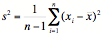The variance has square units, so it is usual to take its square root to obtain the standard deviation, s, which has the same units as the original measurements. The higher the standard deviation s, the mre dispersed the data are around the mean.

Both the variance and standard deviation have values that depend on the measurement scale used. So measuring body weights of newborns in grams will produce much higher variances than if the same newborns were measured in kilograms. To account for the measurement scale, it is typical to use the coefficient of variability (sometimes called the coefficient of variance) which is the standard deviation divided by the arithmetic mean, or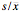, which is dimensionless and has no units. This coefficient of variability is thus independent of the measurement scale used.

The above measures of dispersion all apply to ratio scale data. For nominal scale data, there is no mean or variance that makes sense, but there certainly can be a measure of how spread out the data are amongst the various categories, a concept called diversity. In ecology, this is typically used as a measure of how biologically diverse an area is, in terms not just of how many different species there are (called richness, though in the popular literature this is often just called biodiversity), but how evenly those species are distributed. The system is very uneven if virtually all the individuals found are of one species with only rare individuals in the other species. The system is very even if all species have equal abundances. Here the Shannon-Weaver index is a measure (derived from information theory) of diversity obtained by taking the negative of the mean of the product of the frequency of each species times the logarithm of that frequency. So if we collect observations and find n different species in a region, and the frequency that species i occurs in the data set is fi, then the Shannon-Weaver index is